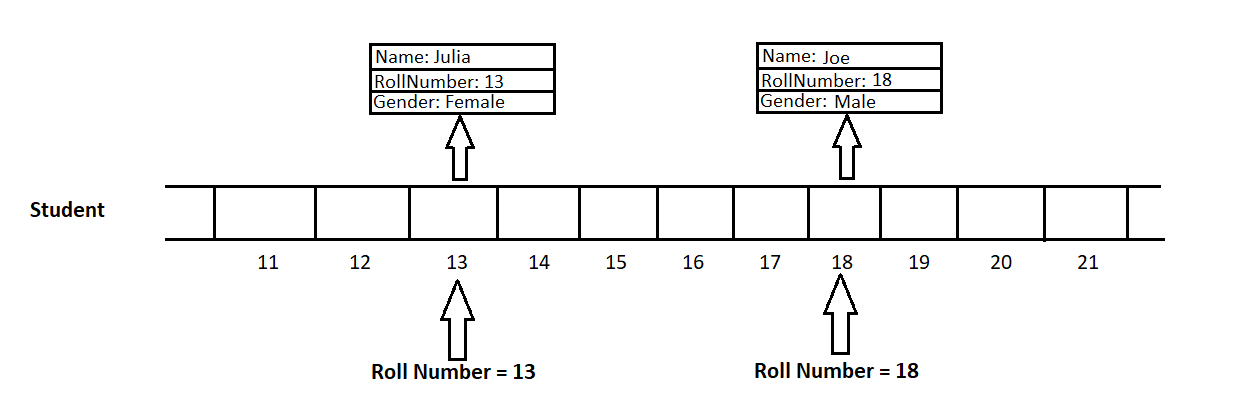Related Articles
Java Program to Implement Direct Addressing Tables
• Difficulty Level : Easy
• Last Updated : 07 Jan, 2021

Data structures are primarily used to store data and manage a large amount of data, and efficient data structures are helpful for developing better algorithms. The main operations performed on a data structure are Insert, Search, Update, and Delete. With increasing amounts of data, all these operations tend to take a large amount of time.

There may be scenarios where we want to have all these operations to be performed in O(1) time i.e. constant time. To implement this, we have a data structure called a Direct Addressing Table.

Direct Addressing Table is a random data structure that maps records with their corresponding unique keys using Arrays. In Direct Address Tables, the keys of records are used directly as indexes. This helps in fast and efficient Insert, Search, and Delete operations.

The following operations can be done in O(1) time:

1. Insertion: Inserting an element at an index in an array takes O(1) time. Here also, for the insertion of an element, the key is used as an index.
2. Searching: Using the key values as the indexes give a faster lookup for searching any record.
3. Deletion: To delete an element from the Direct Address Table, set the index/location to point to null.

Example

Let’s consider we are given the task of creating and maintaining a catalog for data of college students of a particular division with a maximum capability of 70 students per division. Each student has a unique roll number assigned to him/her. The task is to store the complete information of every student efficiently. Now, we can use the roll number of a student as a key to put his/her data at that index. Firstly, we create an array arr of size 70 which will act as our direct address table.

Let’s suppose we insert the student data with roll number 13, we use 13 as an index and insert that student’s data at arr. Insert another student’s data with roll number 18, we use 18 as an index and insert that student’s data at arr. The image below is a representation of the insertion operation being performed.Direct Addressing Table using arrays

Now, consider we want to retrieve the information about the student with roll number 18, we can directly use 18 as an index and get that student’s data at arr. This address table lookup method gives access to data in O(1) time. Suppose we want to delete the information about the student with roll number 13 (maybe because he left the college), use 13 as an index, and remove that student’s data at arr by setting it to null.

But there are some limitations of using this data structure:

1. The keys must be integers / can be converted to integers.
2. All the keys must be unique.
3. The range of integer keys should be relatively small (else we will need a very large amount of memory)
4. The integer keys should be relatively close enough (else there will be more empty slots and memory will be wasted)

Implementation:

Let’s look at the implementation of the above example:

## Java

 `// Java program to implement Direct Addressing Table `` ` `import` `java.util.*;`` ` `class` `Student {``    ``int` `rollNumber;``    ``String name, gender;``   ` `    ``Student(``int` `rollNumber, String name, String gender)``    ``{``        ``this``.rollNumber = rollNumber;``        ``this``.name = name;``        ``this``.gender = gender;``    ``}``}`` ` `class` `DirectAddressingTable {``    ``public` `static` `void` `main(String[] args)``    ``{``        ``// We want to create a catalogue of college students of some division ``          ``// Each division has atmost 70 students and roll number starts from 1 ``        ``// Create "Direct Addressing Table" (catalogue) for the students' data ``       ` `        ``Student[] catalogue = ``new` `Student[``71``];`` ` `        ``// Insert some students' data into the catalogue``        ``insert(catalogue, ``new` `Student(``11``, ``"Rahul"``, ``"Male"``));``        ``insert(catalogue, ``new` `Student(``18``, ``"Joe"``, ``"Male"``));``        ``insert(catalogue, ``new` `Student(``15``, ``"Kavya"``, ``"Female"``));``        ``insert(catalogue, ``new` `Student(``13``, ``"Julia"``, ``"Female"``));`` ` `        ``// if the student with given rollnumber exists,``        ``// then "search" function returns the record``        ``// else returns null``         ` `        ``Student student = search(catalogue, ``18``);``        ``printInformation(``18``, student);`` ` `        ``// delete student record with roll number 36``        ``delete(catalogue, ``13``);``       ` `        ``student = search(catalogue, ``13``);``       ` `        ``// This will print "No student found" as we deleted``        ``// the record``        ``printInformation(``13``, student);``    ``}`` ` `    ``// Insert function to add student in the catalogue``    ``public` `static` `void` `insert(Student[] catalogue,``                              ``Student student)``    ``{``        ``catalogue[student.rollNumber] = student;``    ``}`` ` `    ``// Search function to get the record of student from the``    ``// catalogue``    ``public` `static` `Student search(Student[] catalogue,``                                 ``int` `rollNumber)``    ``{``        ``return` `catalogue[rollNumber];``    ``}`` ` `    ``// Delete function to remove the record of student from``    ``// the catalogue``    ``public` `static` `void` `delete(Student[] catalogue,``                              ``int` `rollNumber)``    ``{``        ``// As we are using arrays, we just will this``        ``// location to point to null``        ``catalogue[rollNumber] = ``null``;``    ``}`` ` `    ``// Function to print student's information``    ``public` `static` `void` `printInformation(``int` `rollNumber,``                                             ``Student student)``    ``{``        ``// print student information if record exists``        ``if` `(student != ``null``)``            ``System.out.println( ``"Student with roll number "` `+ rollNumber``                ``+ ``" - Name: "` `+ student.name``                ``+ ``", Gender: "` `+ student.gender);``        ``else``            ``System.out.println( ``"No student found with roll number "``                ``+ rollNumber);``    ``}``}`
Output
```Student with roll number 18 - Name: Joe, Gender: Male
No student found with roll number 13```

Attention reader! Don’t stop learning now. Get hold of all the important Java Foundation and Collections concepts with the Fundamentals of Java and Java Collections Course at a student-friendly price and become industry ready. To complete your preparation from learning a language to DS Algo and many more,  please refer Complete Interview Preparation Course.

My Personal Notes arrow_drop_up